# Basic Geometry : Plane Geometry

## Example Questions

### Example Question #3 : How To Find An Angle Of A Line

Straight

Acute

Scalene

Right

Obtuse

Obtuse

Explanation:

Obtuse angles are greater than.

Scalene is a designation for triangles that have one angle greater than, but this figure is not a triangle.

Acute angles are less than, right angles are, and straight angles are.

Therefore this angle is obtuse.

### Example Question #4 : How To Find An Angle Of A Line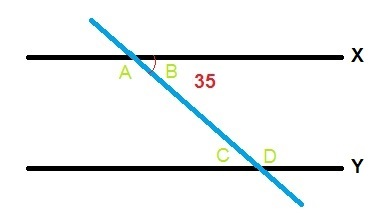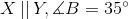What is the measure of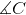?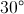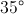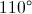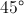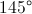Explanation:

When two parallel lines are crossed by a third line (called the transversal), the measure of the angles follows a specific pattern. The pairs of angles inside the two lines and on opposite sides are called alternate interior angles. Alternate interior angles, such as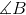and, have the same degree measure. Therefore, the measure ofis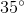.

### Example Question #5 : How To Find An Angle Of A Line

Mark is training for cross country and comes across a new hill to run on. After Mark runsmeters, he's at a height ofmeters. What is the hill's angle of depression when he's at an altitude ofmeters?

The same as the angle of inclination

Cannot be determined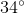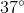Explanation: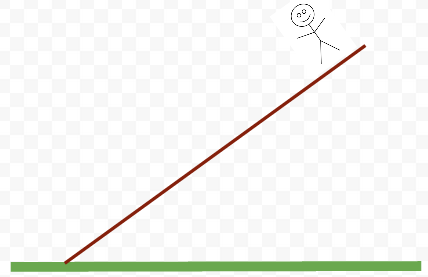Upon reading the question, we're left with this spatial image of Mark in our heads. After adding in the given information, the image becomes more like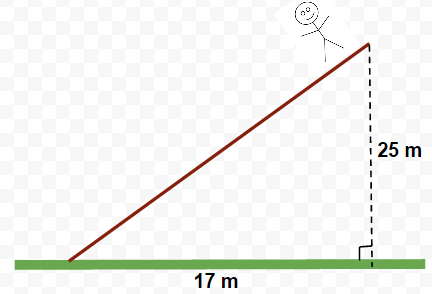The hill Mark is running on can be seen in terms of a right triangle. This problem quickly becomes one that is asking for a mystery angle given that the two legs of the triangle are given. In order to solve for the angle of depression, we have to call upon the principles of the tangent function. Tan, Sin, or Cos are normally used when there is an angle present and the goal is to calculate one of the sides of the triangle. In this case, the circumstances are reversed.

Remember back to "SOH CAH TOA." In this problem, no information is given about the hypotenuse and nor are we trying to calculate the hypotenuse. Therefore, we are left with "TOA." If we were to check, this would work out because the angle at Mark's feet has the information for the opposite side and adjacent side.

Because there's no angle given, we must use the principles behind the tan function while using a fraction composed of the given sides. This problem will be solved using arctan (sometimes denoted as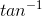).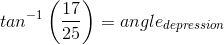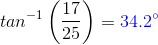### Example Question #6 : How To Find An Angle Of A Line

Two angles are supplementary and have a ratio of 1:4.  What is the size of the smaller angle?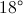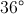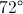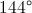Explanation:

Since the angles are supplementary, their sum is 180 degrees.  Because they are in a ratio of 1:4, the following expression could be written: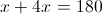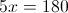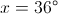### Example Question #7 : How To Find An Angle Of A Line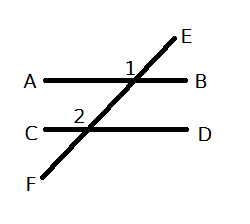AB and CD are two parrellel lines intersected by line EF. If the measure of angle 1 is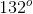, what is the measure of angle 2?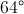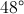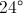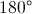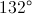Explanation:

The angles are equal. When two parallel lines are intersected by a transversal, the corresponding angles have the same measure.

### Example Question #1 : How To Find An Angle Of A Line

Lines A and B in the diagram below are parallel. The triangle at the bottom of the figure is an isosceles triangle.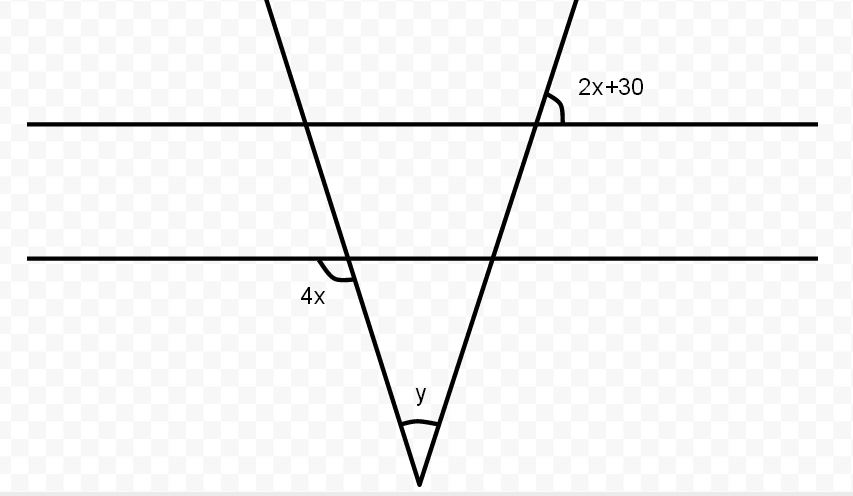What is the degree measure of angle?Explanation:

Since A and B are parallel, and the triangle is isosceles, we can use the supplementary rule for the two angles,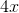and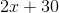which will sum up to. Setting up an algebraic equation for this, we get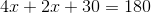. Solving for, we get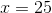. With this, we can get either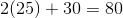(for the smaller angle) or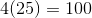(for the larger angle - must then use supplementary rule again for inner smaller angle). Either way, we find that the inner angles at the top are 80 degrees each. Since the sum of the angles within a triangle must equal 180, we can set up the equation as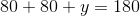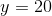degrees.

### Example Question #1 : Plane Geometry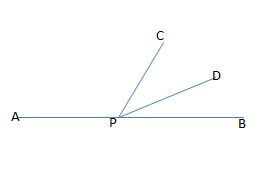Figure not drawn to scale.

In the figure above, APB forms a straight line. If the measure of angle APC is eighty-one degrees larger than the measure of angle DPB, and the measures of angles CPD and DPB are equal, then what is the measure, in degrees, of angle CPB?

33

40

114

66

50

66

Explanation:

Let x equal the measure of angle DPB. Because the measure of angle APC is eighty-one degrees larger than the measure of DPB, we can represent this angle's measure as x + 81. Also, because the measure of angle CPD is equal to the measure of angle DPB, we can represent the measure of CPD as x.

Since APB is a straight line, the sum of the measures of angles DPB, APC, and CPD must all equal 180; therefore, we can write the following equation to find x:

x + (x + 81) + x = 180

Simplify by collecting the x terms.

3x + 81 = 180

Subtract 81 from both sides.

3x = 99

Divide by 3.

x = 33.

This means that the measures of angles DPB and CPD are both equal to 33 degrees. The original question asks us to find the measure of angle CPB, which is equal to the sum of the measures of angles DPB and CPD.

measure of CPB = 33 + 33 = 66.

### Example Question #1 : How To Find An Angle Of A Line

One-half of the measure of the supplement of angle ABC is equal to the twice the measure of angle ABC. What is the measure, in degrees, of the complement of angle ABC?

36

18

54

90

72

54

Explanation:

Let x equal the measure of angle ABC, let y equal the measure of the supplement of angle ABC, and let z equal the measure of the complement of angle ABC.

Because x and y are supplements, the sum of their measures must equal 180. In other words, x + y = 180.

We are told that one-half of the measure of the supplement is equal to twice the measure of ABC. We could write this equation as follows:

(1/2)y = 2x.

Because x + y = 180, we can solve for y in terms of x by subtracting x from both sides. In other words, y = 180 – x. Next, we can substitute this value into the equation (1/2)y = 2x and then solve for x.

(1/2)(180-x) = 2x.

Multiply both sides by 2 to get rid of the fraction.

(180 – x) = 4x.

180 = 5x.

Divide both sides by 5.

x = 36.

The measure of angle ABC is 36 degrees. However, the original question asks us to find the measure of the complement of ABC, which we denoted previously as z. Because the sum of the measure of an angle and the measure of its complement equals 90, we can write the following equation:

x + z = 90.

Now, we can substitute 36 as the value of x and then solve for z.

36 + z = 90.

Subtract 36 from both sides.

z = 54.

### Example Question #3 : Geometry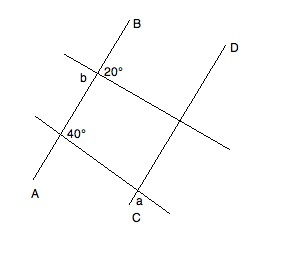In the diagram, AB || CD. What is the value of a+b?

160°

140°

80°

60°

160°

Explanation:

Refer to the following diagram while reading the explanation: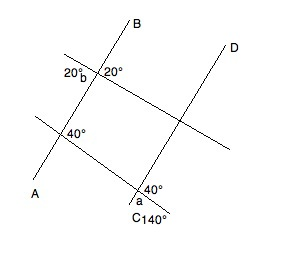We know that angle b has to be equal to its vertical angle (the angle directly "across" the intersection).  Therefore, it is 20°.

Furthermore, given the properties of parallel lines, we know that the supplementary angle to a must be 40°.  Based on the rule for supplements, we know that a + 40° = 180°.  Solving for a, we get a = 140°.

Therefore, a + b = 140° + 20° = 160°

### Example Question #1 : How To Find The Angle Of Two Lines

In rectangle ABCD, both diagonals are drawn and intersect at point E.

Let the measure of angle AEB equal x degrees.

Let the measure of angle BEC equal y degrees.

Let the measure of angle CED equal z degrees.

Find the measure of angle AED in terms of x, y, and/or z.

360 – x + y + z

180 – (x + y + z)

180 – y

180 – 2(x + z)

180 – 1/2(x + z)

180 – 1/2(x + z)

Explanation:

Intersecting lines create two pairs of vertical angles which are congruent. Therefore, we can deduce that y = measure of angle AED.

Furthermore, intersecting lines create adjacent angles that are supplementary (sum to 180 degrees). Therefore, we can deduce that x + y + z + (measure of angle AED) = 360.

Substituting the first equation into the second equation, we get

x + (measure of angle AED) + z + (measure of angle AED) = 360

2(measure of angle AED) + x + z = 360

2(measure of angle AED) = 360 – (x + z)

Divide by two and get:

measure of angle AED = 180 – 1/2(x + z)

### All Basic Geometry Resources